# Placing Fibonacci Lines on MT4

The fifth option on the MT4 stock indexes trading platform insert menu is Fibonacci Lines. The Fibonacci Lines Button has the following sub menu buttons shown below: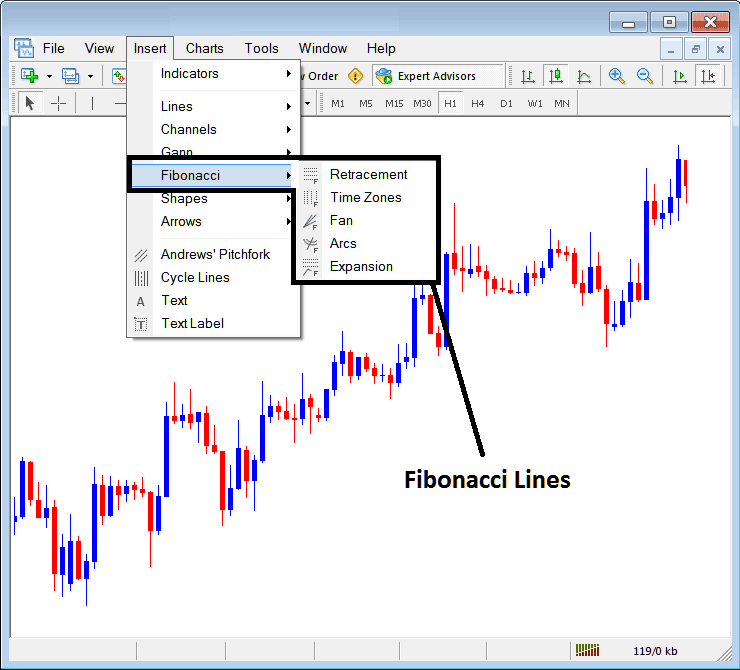Fibonacci Stock Indexes on MT4

## Fibonacci Lines

2. Fibonacci Time Zones

3. Fibonacci Fan

4. Fibonacci Arcs

The Fibonacci retracement can be placed on the a Indices chart from the Fibonacci retracement button. Fibonacci retracement is used to estimate where a indices price retracement is likely to reach. The Fibonacci retracement levels are drawn as shown below. To learn how to draw and use Indices Trading Fibonacci Retracement Levels read the tutorial: How to draw Indices Trading Fibonacci Retracement Levels: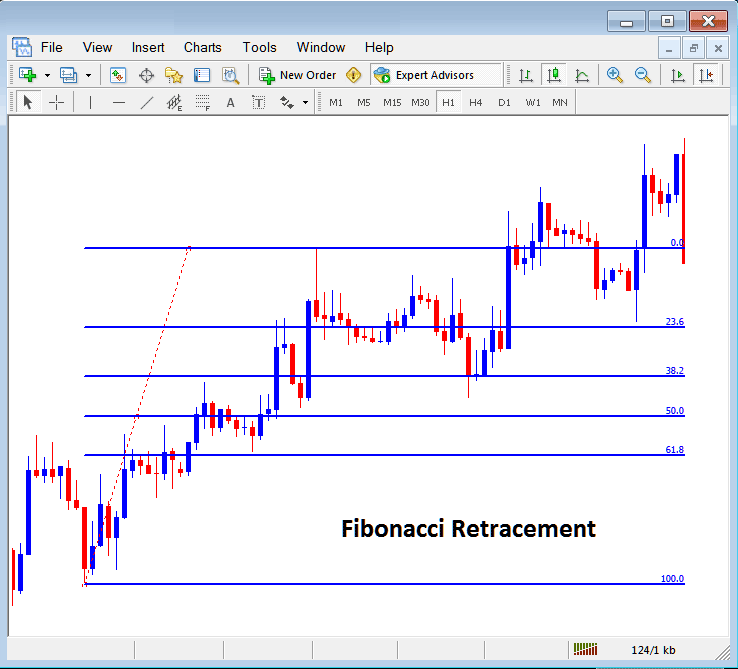Indices Trading Fibonacci Retracement Levels Drawn on a Indices Trading Chart on The MT4 Indices Trading Platform

## Fibonacci Time Zones

The Fibonacci time zones are Fibonacci lines drawn at different Fibonacci timelines, these Fibonacci time zones are calculated using the Fibonacci points 1, 2, 3, 5, 8, 13, 21, 34. The Fibonacci levels are 1+2=3, 2+3=5, 3+5=8, first number add to the next, the next added to the second and so on.

The Fibonacci time zones are shown below, at these time lines stock indexes price changes are likely to occur based on technical analysis of Fibonacci time zones: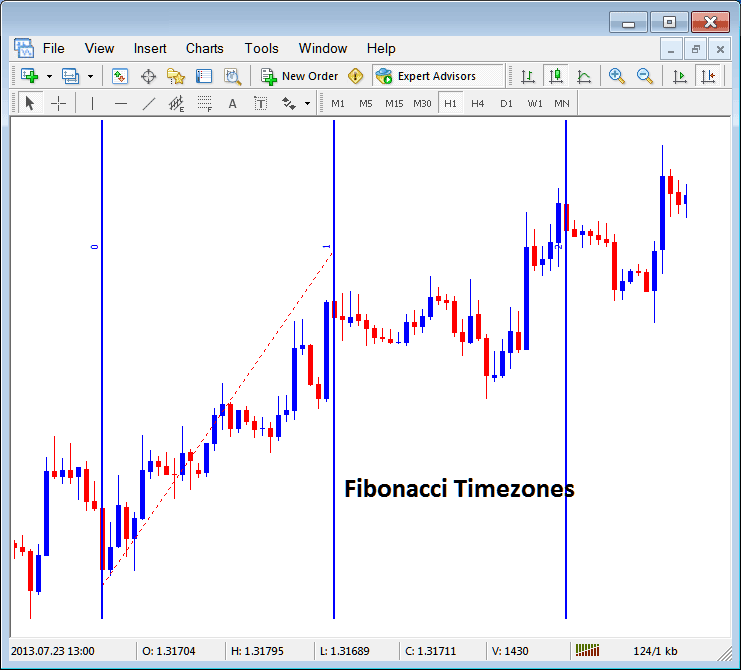Fibonacci Time Zones on MetaTrader 4## Fibonacci Fan

The Fibonacci fan is drawn between two chart points and then three additional lines below the indices trend are drawn as the fans, these are 38.2%, 50.0% and 61.8% Fibonacci retracement levels. The stock indexes price is likely to bounce and continue in the original direction after stock indexes price retraces to any of these fan lines.

The Fibonacci Fan Lines are drawn as shown below: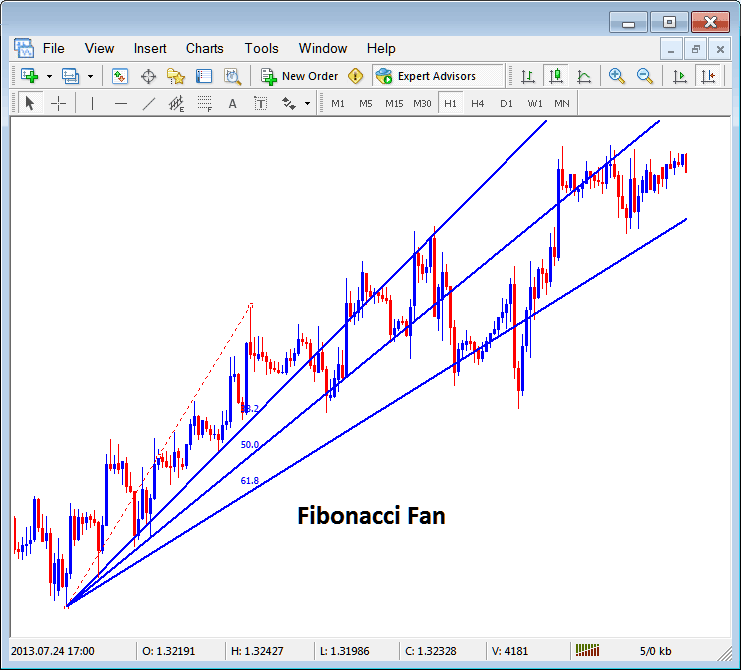Fibonacci Fans on MT4

## Fibonacci Arcs

Fibonacci arcs are drawn using two chart points, the arcs are then drawn as shown below and these arcs represent 38.2%, 50.0% and 61.8% Fibonacci retracement levels.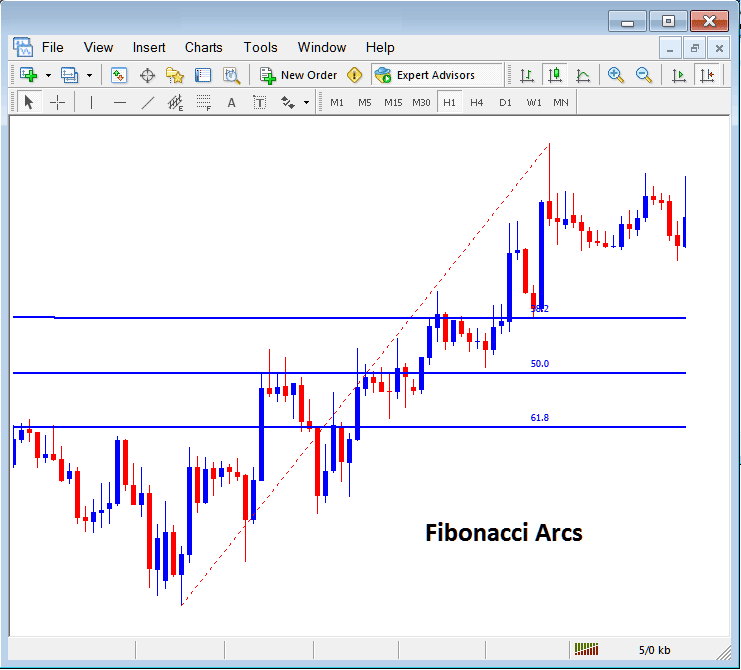Fibonacci Arcs Representing Indices Trading Fibonacci Retracement Zones on a Indices Trading Chart

The Fibonacci expansion is used to determine the most probable level at which traders should set their take profit levels based on Fibonacci expansion level 61.8% and 100.0% expansion levels. The Fibonacci expansion levels are also referred to as Indices Trading Fibonacci Extension Levels or Fibonacci Projection Levels by some traders and these Indices Trading Fibonacci Extension levels are drawn as shown below. Tutorial: How to Draw Indices Trading Fibonacci Expansion Levels: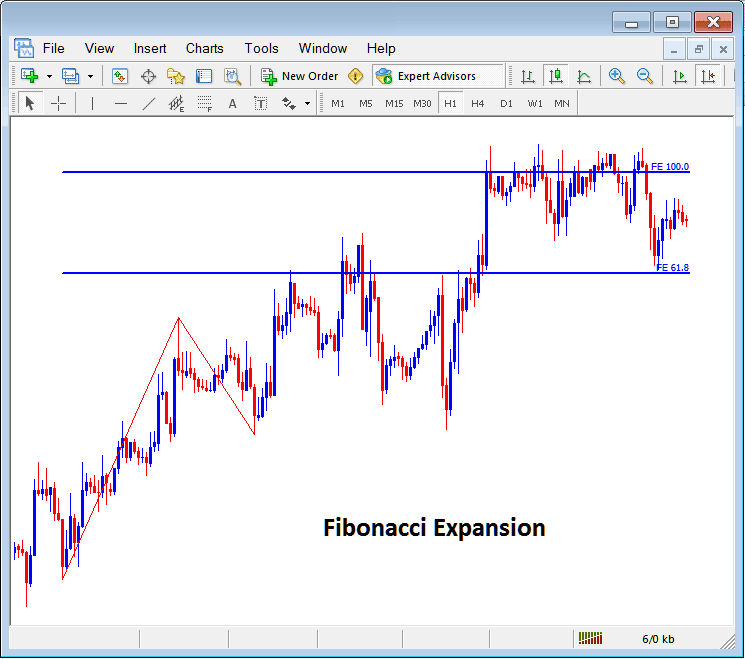How to draw Fibonacci Lines and other Fibonacci studies like Indices Trading Fibonacci Expansion and Retracement on MT4